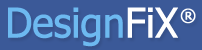Select Page

In a narrow and thin component the resistance for concrete edge failure does not increase with increasing edge distance c1. What is the reason for this illogical baviour?

With edge distances c2,1 and c2,2 and a component thickness h smaller than 1.5 times the edge distance c1, the idealized area Ac,V is truncated by both side edges and the lower edge of the component (see Figure 1). The component will break at the position of the anchors (Figure 1a), the fracture surface is constant and thus, the resistance for concrete edge failure is independent of the edge distance c1. ETAG 001, Annex C, Section 5.2.3.4 considers this under ‘Special cases’. The edge distance c1 is substituted by a virtual edge distance c’1 = max{c2,1/1,5; c2,2/1,5; h/1,5}. With this virtual edge distance, the idealized fracture surface just touches the side edges or the lower edge of the component. If this ‘Special case’ is not applied and the design is carried out for the real edge distance c1 then the resistance decreases with increasing edge distance c1. This is illogical.

If at least one side edge distance or the component thickness is larger than 1.5 times the edge distance c1 then the fracture surface can develop completely into this direction and the resistance for concrete edge failure increases or decreases with increasing or decreasing edge distance c1.

If no front edge distance c1 is present (Figure 1b) then DesignFiX assumes that the concrete component is supported and no edge failure can occur. In this case a side edge failure may be expected (Figure 1b).

Figure 1:
a) Special case„narrow thin component“ (c2,1 < 1,5 · c1; c2,2 < 1,5 · c1; h < 1,5 · c1)
b) Component, supported on two edges (rear and front edge)## DesignFiX - Anchor Design